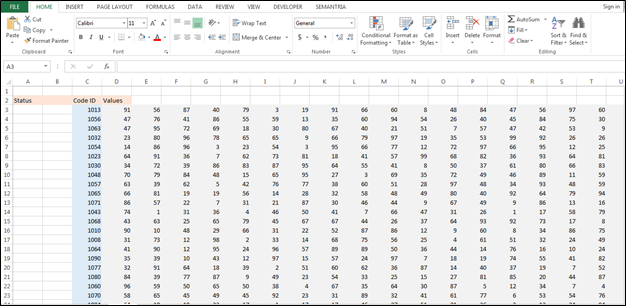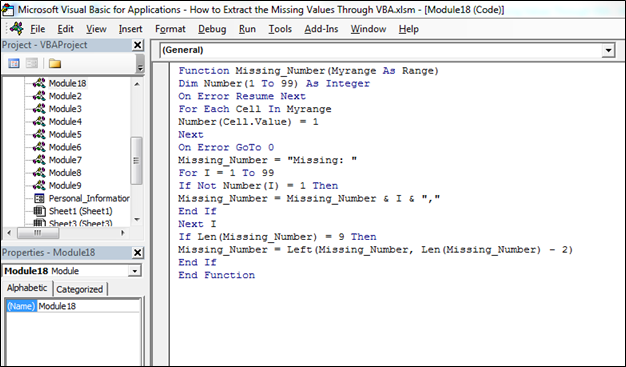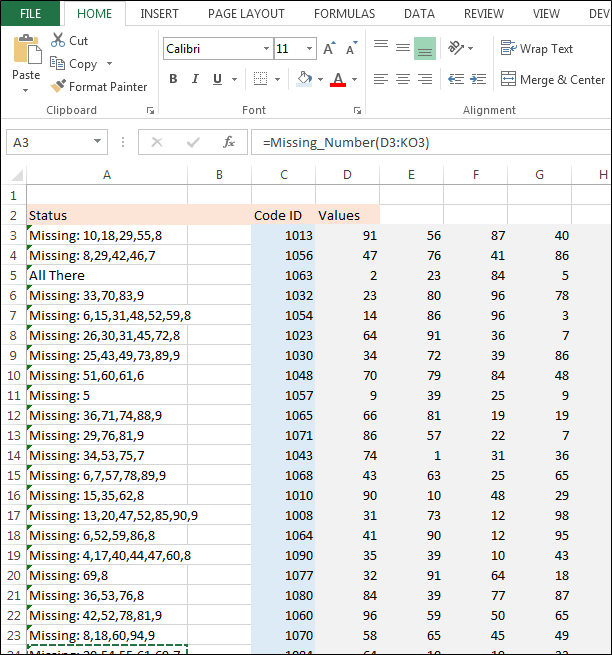# How to Extract the Missing Values through VBA

In this article, we are going to create user defined function to find out the missing number in a range through VBA in Microsoft Excel.

User Defined Function: Microsoft Excel allows you to create your own function as per the requirement, we call it User Defined Function. And, we can use User Defined functions like other functions work in Excel.

Let’s take an example to understand:-

We have a data in which we have values for every code ID, and we want to check the missing value in between 1 to 99, if in the range values are missing then which values all missing and if all values are available then result should be all values are available.We will write the VBA code to find out the missing numbers follow below given steps:

• Open VBA Page press the key Alt+F11
• Insert a module
• Then go to again insert menu and click on procedure, where you will get the procedure if you don’t know how should be start the macro
• Write the below mentioned code:

```Function Missing_Number(Myrange As Range)

Dim Number(1 To 99) As Integer

On Error Resume Next

For Each Cell In Myrange

Number(Cell.Value) = 1

Next

On Error GoTo 0

Missing_Number = "Missing: "

For I = 1 To 99

If Not Number(I) = 1 Then

Missing_Number = Missing_Number & I & ","

End If

Next I

If Len(Missing_Number) = 9 Then

Missing_Number = Left(Missing_Number, Len(Missing_Number) - 2)

End If

End Function
```• Press the key F5 on the keyboard
• Use this macro function as formula
• =Missing_Number(D3:KO3) press enter on the keyboardIn this way, we can return the missing values from the data for every row by using VBA in Microsoft Excel